Lakhmir Singh & Manjit Kaur: Motion, Solutions- 3

# Lakhmir Singh & Manjit Kaur: Motion, Solutions- 3 - Science Class 9

Page No:39

Question 1:
(a) What remains constant in uniform circular motion ?
(b) What changes continuously in uniform circular motion ? ‘

Solution :
(a)Speed
(b)Direction of motion

Question 2:
State whether the following statement is true or false :
Earth moves round the sun with uniform velocity.

Solution :
No, earth moves round the sun with uniform speed, but its velocity changes continuously.

Question 3:
A body goes round the sun with constant speed in a circular orbit. Is the motion uniform or accelerated ?
Solution :
The motion is accelerated.

Question 4:
What conclusion can you draw about the velocity of a body from the displacement-time graph shown below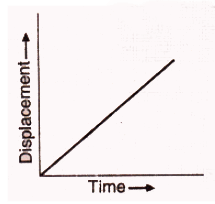Solution :
It represents uniform velocity.

Question 5:
Name the quantity which is measured by the area occupied under the velocity-time graph.
Solution :
Distance travelled by the moving body .

Question 6:
What does the slope of a speed-time graph indicate ?
Solution :
The slope of a speed-time graph indicates acceleration.

Question 7:
What does the slope of a distance-time graph indicate ?
Solution :
The slope of a distance-time graph indicates speed.

Question 8:
Give one example of a motion where an object does not change its speed but its direction of motion changes continuously.
Solution :
Motion of moon around the earth.

Question 9:
Name the type of motion in which a body has a constant speed but not constant velocity.
Solution :
Uniform circular motion.

Question 10:
What can you say about the motion of a body if its speed-time graph is a straight line parallel to the time axis ?
Solution :
The Speed of the body is constant or uniform.

Page No:40

Question 11:
What conclusion can you draw about the speed of a body from the following distance-time graph ?Solution :
The body has uniform speed.

Question 12:
What can you say about the motion of a body whose distance-time graph is a straight line parallel to the time axis ?
Solution :
The body is not moving. It is stationary.

Question 13:
What conclusion can you draw about the acceleration of a body from the speed-time graph shown below ?Solution :
It represents non-uniform acceleration.

Question 14:
A satellite goes round the earth in a circular orbit with constant speed. Is the motion uniform or accelerated ?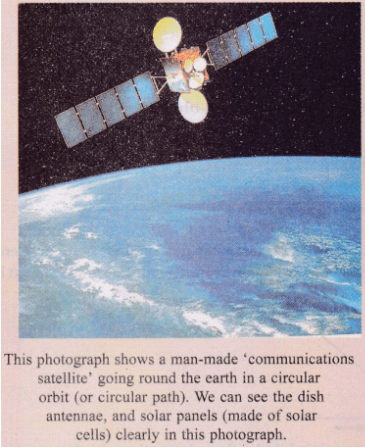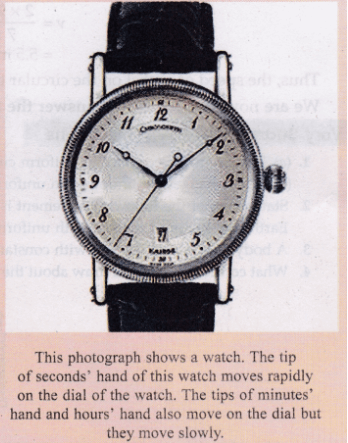Solution :
It is accelerated motion as the velocity is changing continuously.

Question 15:
What type of motion is represented by the tip of the ‘seconds’ hand’ of a watch ? Is it uniform or accelerated ?
Solution :
The tip of the ‘seconds’ hand’ of a watch represents uniform circular motion. It is an accelerated motion.

Question 16:
Fill in the following blanks with suitable words :
(a) If a body moves with uniform velocity, its acceleration is…………………………..
(b) The slope of a distance-time graph indicates………………….. of a moving body.
(c) The slope of a speed-time graph of a moving body gives its………………………..
(d) In a speed-time graph, the area enclosed by the speed-time curve and the time axis gives the…………….. by the body.
(e) It is possible for something to accelerate but not change its speed if it moves in a………………………..

Solution :
(a) zero
(b) speed
(c) acceleration
(d) distance travelled
(e) circular path

Page No:41

Question 17:
Is the uniform circular motion accelerated ? Give reasons for your answer
Solution :
Yes, uniform circular motion is accelerated because the velocity changes due to continuous change in the direction of motion.

Question 18:
Write the formula to calculate the speed of a body moving along a circular path. Give the meaning of each symbol which occurs in it.
Solution :
The speed of a body moving along a circular path is given by the formula: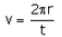where, v= speed

π=3.14 ( it is a constant)
r = radius of circular path
t= time taken for one round of circular path

Question 19:
Explain why, the motion of a body which is moving with constant speed in a circular path is said to be accelerated.
Solution :
The motion of a body which is moving with constant speed in a circular path is said to be accelerated because its velocity changes continuously due to the continuous change in the direction of motion.

Question 20:
What is the difference between uniform linear motion and uniform circular motion ? Explain with examples.
Solution :
Uniform linear motion is uniform motion along a linear path or a straight line. T he direction of motion is fixed. So, it is not accelerated. For e.g.: a car running with uniform speed of 10km/hr on a straight road.
Uniform circular motion is uniform motion along a circular path. T he direction of motion changes continuously. So, it is accelerated. For e.g.: motion of earth around the sun.

Question 21:
State an important characteristic of uniform circular motion. Name the force which brings about uniform circular motion.
Solution :
An important characteristic of uniform circular motion is that the direction of motion in it changes continuously with time, so it is accelerated.
Centripetal force brings about uniform circular motion.

Question 22:
Find the initial velocity of a car which is stopped in 10 seconds by applying brakes. The retardation due to brakes is 2.5 m/s2.
Solution :
Initial velocity, u=?
Final velocity, v=0m/s (car is stopped)
Retardation, a=-2.5 m/s2
Time, t=10s
v=u + at
0=u +(-2.5)x 10
u=25m/s

Question 23:
Describe the motion of a body which is accelerating at a constant rate of 10 m s2. If the body starts from rest, how much distance will it cover in 2 s ?
Solution :
The velocity of this body is increasing at a rate of ’10 metres per second’ every second.
Initial velocity, u=0m/s
Time, t=2s
Acceleration, a=10m/s2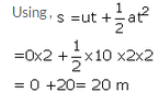Question 24:
A motorcycle moving with a speed of 5 m/s is subjected to an acceleration of 0.2 m/s2. Calculate the speed of the motorcycle after 10 seconds, and the distance travelled in this time.
Solution :
Initial velocity, u=5m/s
Final velocity, v=?
Acceleration, a=0.2m/s2
Time, t=10 sec
Using , v=u + at
v=5 + 0.2 x 10
v=5 + 2 =7 m/s
Now distance travelled in time is calculated;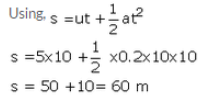Question 25:
A bus running at a speed of 18 km/h is stopped in 2.5 seconds by applying brakes. Calculate the retardation produced.
Solution :
Initial velocity, u=18km/h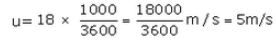Final velocity, v=0m/s
Time, t=2.5 sec
Acceleration, a=?
Using , v= u + at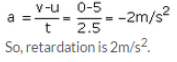Question 26:
A train starting from rest moves with a uniform acceleration of 0.2 m/s2 for 5 minutes. Calculate the speed acquired and the distance travelled in this time.
Solution :
Initial velocity, u=0m/s
Final velocity, v=?
Acceleration, a=0.2 m/s2
Time, t=5min= 5 x60=300 sec
Using, v = u + at
v =0 + 0.2 x 300=60m/s
And the distance travelled is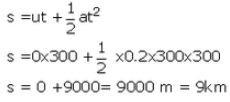Question 27:
Name the two quantities, the slope of whose graph gives :
(a) speed, and
(b) acceleration

Solution :
(a) Distance and Time
(b) Speed (or velocity) and Time

Question 28:
A cheetah starts from, rest, and accelerates at 2 m/s2 for 10 seconds. Calculate :
(a) the final velocity
(b) the distance travelled.

Solution :
Initial velocity,u=0 m/s
Final velocity, v=?
Acceleration, a=2 m/s2
Time, t=10s
(a)Using,
v = u + at
v=0 + 2 x 10= 20 m/s
(b)Distance travelled is: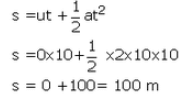Question 29:
A train travelling at 20 m s-1 accelerates at 0.5 m s-2 for 30 s. How far will it travel in this time ?
Solution :
Initial velocity, u=20m/s
Time, t=30 s
Acceleration,
a=0.5m/s2
Distance travelled is: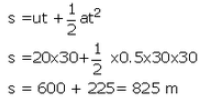Question 30:
A cyclist is travelling at 15 m s-1. She applies brakes so that she does not collide with a wall 18 m away. What deceleration must she have ?
Solution :
Initial velocity, u=15m/s
Final velocity, v=0m/s
Distance, s=18m
Acceleration, a=?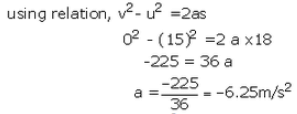So, deceleration is 6.25 m/s2

Question 31:
Draw a velocity-time graph to show the following motion :
A car accelerates uniformly from rest for 5 s ; then it travels at a steady’ velocity for 5 s.
Solution :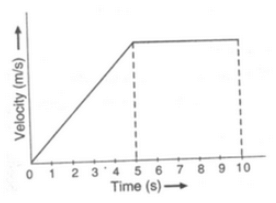Question 32:
The velocity-time graph for part of a train journey is a horizontal straight line. What does this tell you about
(a) the train’s velocity, and (b) about its acceleration ?

Solution :
(a) The train has a uniform velocity.
(b) There is no acceleration.

Question 33:
(a) Explain the meaning of the following equation of motion :v = u + at, where symbols have their usual meanings.
(b) A body starting from rest travels with uniform acceleration. If it travels 100 m in 5 s, what is the value of acceleration ?

Solution :
(a) v=u + at is the first equation of motion. It gives the velocity acquired by a body in time t when the body has initial velocity u and uniform acceleration a.
(b)Initial velocity, u=0m/s
Time, t=5 s
Distance, s=100m
Acceleration, a=?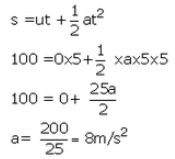Question 34:
(a) Derive the formula : v = u + at, where the symbols have usual meanings.
(b) A bus was moving with a speed of 54 km/h. On applying brakes it stopped in 8 seconds. Calculate the acceleration.
Solution :
(a) Consider a body having initial velocity ‘u’. Suppose it is subjected to a uniform acceleration ‘a’ so that after time’t’ its final velocity becomes ‘v’. Now, from the definition of acceleration we know that:
(b) Initial velocity, u=54km/h= 15m/s
Final velocity, v=0m/s
Time, t=8s
Acceleration, a=?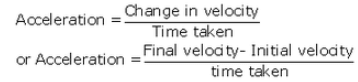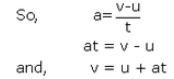where v= final velocity of the body

u = initial velocity of the body

a = acceleration

and t= time taken

(b) initial velocity , u = 54 km/h = 15m/s

Final velocity, v = 0 m/s

Time, t = 8s

Acceleration a= ?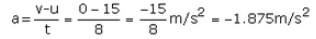Question 35:
(a) Derive the formula :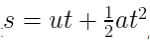, where the symbols have usual meanings.
(b) A train starting from stationary position and moving with uniform acceleration attains a speed of 36 km per hour in 10 minutes. Find its acceleration.
Solution :
(a) Suppose a body has an initial velocity ‘u’ and a uniform acceleration’ a’ for time ‘t’ so that its final velocity becomes ‘v’. Let the distance travelled by the body in this time be ‘s’. The distance travelled by a moving body in time ‘t’ can be found out by considering its average velocity. Since the initial velocity of the body is ‘u’ and its final velocity is ‘v’, the average velocity is given by:
(b) Initial velocity, u=0m/s

Final velocity, v=36km/h=10m/s
Time, t=10min =10 x 60=600 sec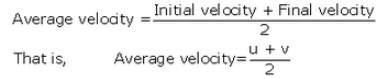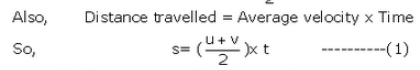From the first equation of motion, we have v= u+at

Put the value of v in equation (1), we get: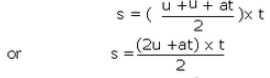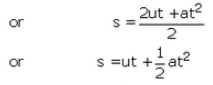Where s = distance travelled by the body in time t

u = initial velocity of the body

and a = acceleration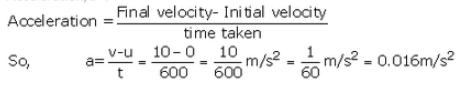Question 36:
(a) Write the three equations of uniformly accelerated motion. Give the meaning of each symbol which occurs in them.
(b) A car acquires a velocity of 72 km per hour in 10 seconds starting from rest. Find
(i) the acceleration,
(ii) the average velocity, and
(iii) the distance travelled in this time.
Solution :

(b) Initial Velocity , u = 0 m/s

Final velocity, v = 72km/h = 20m/s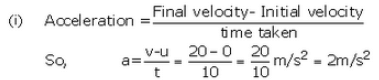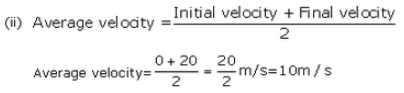(iii) Distance travelled = Average velocity x time

= 10 m/s  x 10 sec = 100m

Question 37:
(a) What is meant by uniform circular motion ? Give two examples of uniform circular motion.
(b) The tip of seconds’ hand of a dock takes 60 seconds to move once on the circular dial of the clock. If the radius of the dial of the clock be 10.5 cm, calculate the speed of the tip of the seconds’ hand of the clock. (Given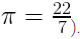Solution :
(a) When a body moves in a circular path with uniform speed (constant speed), its motion is called uniform circular motion. For e.g.
(i) Artificial satellites move in uniform circular motion around the earth.
(ii) Motion of a cyclist on a circular track.
(b) The speed of a body moving along a circular path is given by the formula: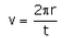Given, t=60 sec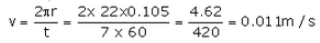The document Lakhmir Singh & Manjit Kaur: Motion, Solutions- 3 | Science Class 9 is a part of the Class 9 Course Science Class 9.
All you need of Class 9 at this link: Class 9

## Science Class 9

66 videos|355 docs|97 tests

## FAQs on Lakhmir Singh & Manjit Kaur: Motion, Solutions- 3 - Science Class 9

 1. What is motion?Ans. Motion is defined as the change in position of an object with respect to its surroundings over time. It can be described in terms of distance, displacement, speed, velocity, and acceleration.
 2. What are the different types of motion?Ans. There are three main types of motion: 1. Translational motion: When an object moves along a straight line, it is called translational motion. 2. Rotational motion: When an object spins or rotates about a fixed axis, it is called rotational motion. 3. Vibrational motion: When an object oscillates back and forth around a fixed position, it is called vibrational motion.
 3. What is the difference between speed and velocity?Ans. Speed and velocity both describe how fast an object is moving, but they are different concepts. Speed is a scalar quantity and only measures the magnitude of the object's motion. On the other hand, velocity is a vector quantity that not only measures the magnitude but also includes the direction of motion.
 4. How is acceleration related to motion?Ans. Acceleration is the rate at which an object's velocity changes over time. If an object speeds up, slows down, or changes direction, it is experiencing acceleration. It is directly related to motion as it quantifies the change in velocity of an object.
 5. What are the SI units of motion-related quantities?Ans. The SI (International System of Units) units for motion-related quantities are as follows: - Distance: meter (m) - Displacement: meter (m) - Speed: meter per second (m/s) - Velocity: meter per second (m/s) - Acceleration: meter per second squared (m/s²)

## Science Class 9

66 videos|355 docs|97 testsExplore Courses for Class 9 examSignup to see your scores go up within 7 days! Learn & Practice with 1000+ FREE Notes, Videos & Tests.
10M+ students study on EduRev
Track your progress, build streaks, highlight & save important lessons and more!
Related Searches

,

,

,

,

,

,

,

,

,

,

,

,

,

,

,

,

,

,

,

,

,

,

,

,

;